Paul's Online Notes
Home / Calculus I / Applications of Derivatives / More Optimization Problems
Show Mobile Notice Show All Notes Hide All Notes
Mobile Notice
You appear to be on a device with a "narrow" screen width (i.e. you are probably on a mobile phone). Due to the nature of the mathematics on this site it is best views in landscape mode. If your device is not in landscape mode many of the equations will run off the side of your device (should be able to scroll to see them) and some of the menu items will be cut off due to the narrow screen width.

### Section 4.9 : More Optimization

1. We want to construct a window whose middle is a rectangle and the top and bottom of the window are semi-circles. If we have 50 meters of framing material what are the dimensions of the window that will let in the most light?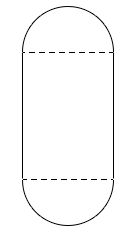Solution
2. Determine the area of the largest rectangle that can be inscribed in a circle of radius 1.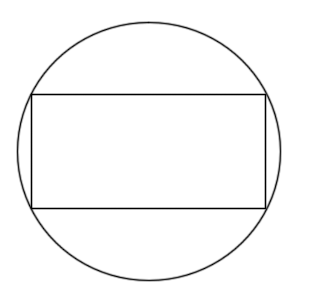Solution
3. Find the point(s) on $$x = 3 - 2{y^2}$$ that are closest to $$\left( { - 4,0} \right)$$. Solution
4. An 80 cm piece of wire is cut into two pieces. One piece is bent into an equilateral triangle and the other will be bent into a rectangle with one side 4 times the length of the other side. Determine where, if anywhere, the wire should be cut to maximize the area enclosed by the two figures. Solution
5. A line through the point $$\left( {2,5} \right)$$ forms a right triangle with the $$x$$-axis and $$y$$-axis in the 1st quadrant. Determine the equation of the line that will minimize the area of this triangle.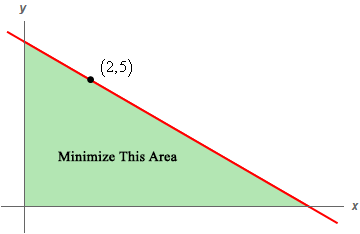Solution
6. A piece of pipe is being carried down a hallway that is 18 feet wide. At the end of the hallway there is a right-angled turn and the hallway narrows down to 12 feet wide. What is the longest pipe (always keeping it horizontal) that can be carried around the turn in the hallway?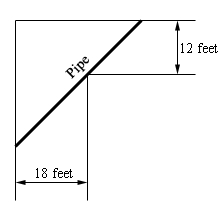Solution
7. Two 10 meter tall poles are 30 meters apart. A length of wire is attached to the top of each pole and it is staked to the ground somewhere between the two poles. Where should the wire be staked so that the minimum amount of wire is used?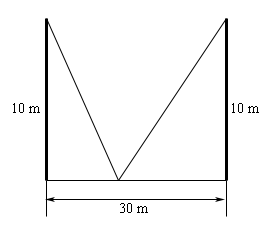Solution